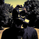# Indicators: AccSwingIndex, ASI Oscillator and RangeExpansionInde

8193 görüntülenme
8193
Accumulation Swing Index
-----------------------------------------------
ASI is a cumulative sum of swing index , developed by Wilder. ASI attemps to show the "real market" by quantifying price. This allows the use of classic support/resistance analysis on the index itself. Typical analysis involves looking for breakouts, new highs and lows, and divergences.

Wilder notes the following characteristics of the Accumulation Swing Index:
- It provides a numerical value that quantifies price swings.
- It defines short-term swing points.
- It indicates the real strength and direction of the market.

Use this for confirmation on S/R breaks, levels et al.

- http://www.investopedia.com/articles/tec...

ASI Oscillator
-----------------------------------------------
I converted the ASI in to an oscillator. Advantage over plain ASI is it points out clearly the accumulation drops (distribution) and divergences. Disadvantage is you won't be able to do normal S/R, channel analysis.

Since this is my own concoction, there is no published theory. Try this out to find out its usecases/nuances :)

Range Expansion Index
-----------------------------------------------
Developed by Tom Demark (first discussed in his book "New Science of Technical Analysis"), this indicator plots the relation between the sum of strong price changes to all price changes for any given period.

Excellent discussion on setups using REI:

http://en.wikipedia.org/wiki/Range_expan...
```//
// @author LazyBear
// If you use this code in its orignal/modified form, do drop me a note.
//
study("Accumulation Swing Index [LazyBear]", shorttitle="ASI_LB")
limit = input(30, "Limit")
lengthMA=input(8, "SMA Length")
showMA=input(true, type=bool)

swingindex( limit ) =>
r1 = abs( high - close )
r2 = abs( low - close )
r3 = abs( high - low )
r4 = abs( close - open )
k = max( r1, r2 )
r = iff( r1 >= max( r2, r3 ), r1 - r2/2 + r4/4,
iff( r2 >= max( r1, r3 ), r2 - r1/2 + r4/4,
r3 + r4/4 ) )
iff( r == 0, 0, 50 * ( ( close - close + 0.5 * ( close - open ) + 0.25 * ( close - open ) ) / r ) * k/limit )

calc_asi( limit ) =>
cum( swingindex( limit ) )

asi=calc_asi( limit )
s=sma(asi,lengthMA)

plot(asi , color=red, linewidth=2)
plot(showMA?sma(asi, lengthMA):na, color=teal)```@LazyBear , I can see the REI when I add this script. Can you please share a script that only displays the REI. Thanks for your time.
Cevap Gönder